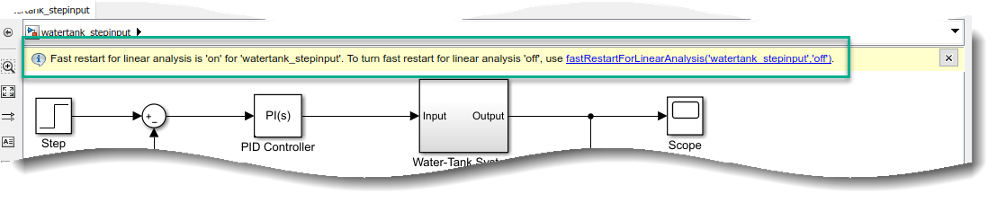Documentation

### This is machine translation

Mouseover text to see original. Click the button below to return to the English version of the page.

Note: This page has been translated by MathWorks. Click here to see
To view all translated materials including this page, select Country from the country navigator on the bottom of this page.

# fastRestartForLinearAnalysis

Fast restart for linear analysis

## Syntax

``fastRestartForLinearAnalysis(model,'on')``
``fastRestartForLinearAnalysis(model,'on',Name,Value)``
``fastRestartForLinearAnalysis(model,'off')``

## Description

example

````fastRestartForLinearAnalysis(model,'on')` prepares the `model` for single compilation workflows by turning fast restart for linear analysis `'on'`. Once a compiling function is called, the model will remain compiled after the function is finished executing. Compiling functions can then be later called without any additional compilations. If the linear analysis points or block substitutions change with subsequent calls to compiling functions, the model is recompiled.```

example

````fastRestartForLinearAnalysis(model,'on',Name,Value)` prepares the `model` for single compilation workflows with additional options specified by one or more `Name,Value` pair arguments.```

example

````fastRestartForLinearAnalysis(model,'off')` turns fast restart for linear analysis off and restores the model parameters to their original value. Simulink® does not let you close the `model` while it is in a complied state. Use this syntax to turn fast restart for linear analysis off to close the `model`.You can also click the link at appears on the top of the compiled Simulink model to turn `fastRestartForLinearAnalysis` off. For more information, see Tips.```

## Examples

collapse all

In this example, you will trim and linearize a closed loop engine speed control model with `fastRestartForLinearAnalysis`.

Open the engine speed control model and get the analysis points for linearization. This is done to prevent re-compilation between the first call to `findop` and `linearize`.

```model = 'scdspeedctrl'; open_system(model); io = getlinio(model); fopt = findopOptions('DisplayReport','off');```

Configure the PI controller to use the base workspace variables `kp` and `ki`.

```block = [model,'/PID Controller']; set_param(block,'P','kp'); set_param(block,'I','ki');```

Create a grid of parameters to vary.

```vp = 0.0005:0.0005:0.003; vi = 0.0025:0.0005:0.005; [KP,KI] = ndgrid(vp,vi); N = numel(KP); sz = size(KP);```

Initialize the base workspace variables kp and ki.

```kp = KP(1); ki = KI(1);```

Turn `fastRestartForLinearAnalysis` on and use `io` as `'AnalysisPoints'`.

`fastRestartForLinearAnalysis(model,'on','AnalysisPoints',io);`

Perform the linear analysis in a loop. Make sure the block initialization is called after the parameters are updated when the model is in a compiled state.

```ops = operspec(model); % operating point specifications for i = N:-1:1 kp = KP(i); ki = KI(i); Simulink.Block.eval(block); % evaluate the block op = findop(model,ops,fopt); % trim the model [j,k] = ind2sub(sz,i); sysFastRestartLoop(:,:,j,k) = linearize(model,io,op); % linearize the model end```

Turn off `fastRestartForLinearAnalysis` and close the model.

```fastRestartForLinearAnalysis(model,'off'); bdclose(model);```

## Input Arguments

collapse all

Simulink model name, specified as a character vector, string, `slTuner`, or `slLinearizer` object. The model must be in the current working folder or on the MATLAB® path.

### Name-Value Pair Arguments

Specify optional comma-separated pairs of `Name,Value` arguments. `Name` is the argument name and `Value` is the corresponding value. `Name` must appear inside quotes. You can specify several name and value pair arguments in any order as `Name1,Value1,...,NameN,ValueN`.

Example: `...,'UseBusSignalLabels','on'`

Analysis point set that contains inputs, outputs, and openings, specified as the comma-separated pair consisting of '`AnalysisPoints`' and a linearization I/O object or a vector of linearization I/O objects. To create '`AnalysisPoints`':

• Define the inputs, outputs, and openings using `linio`.

• If the inputs, outputs, and openings are specified in the Simulink model, extract these points from the model using `getlinio`.

Each linearization I/O object in '`AnalysisPoints`' must correspond to the Simulink model `model` or some normal mode model reference in the model hierarchy.

If the linear analysis points change with subsequent calls to compiling functions, the model is recompiled.

For more information on specifying linearization inputs, outputs, and openings, see Specify Portion of Model to Linearize.

Substitute linearizations for blocks and model subsystems, specified as the comma-separated pair consisting of '`BlockSubstitutions`' and one of the following:

• A structure array

• A string array

• A character vector

• A cell array of character vectors

Use '`BlockSubstitutions`' to specify a custom linearization for a block or subsystem. For example, you can specify linearizations for blocks that do not have analytic linearizations, such as blocks with discontinuities or triggered subsystems.

If the block substitutions change with subsequent calls to compiling functions, the model is recompiled.

Flag indicating whether to use bus signal channel numbers or names to label the I/Os in the linearized model, specified as the comma-separated pair consisting of '`UseBusSignalLabels`' and one of the following:

• `'off'` — Use bus signal channel numbers to label I/Os on bus signals in the linearized model.

• `'on'` — Use bus signal names to label I/Os on bus signals in the linearized model. Bus signal names appear in the results when the I/O points are at the output of the following blocks:

• Root-level inport block containing a bus object

• Bus creator block

• Subsystem block whose source traces back to the output of a bus creator block

• Subsystem block whose source traces back to a root-level inport by passing through only virtual or nonvirtual subsystem boundaries

## Tips

Simulink does not let you close the `model` while it is in a complied state. Turn fast restart for linear analysis off to close the `model`. You can turn off `fastRestartForLinearAnalysis` in one of the following ways:

• Use the syntax `fastRestartForLinearAnalysis(model,'off')`.

• Click the link at appears on the top of the Simulink model.## See Also

#### Introduced in R2019a

##### SupportGet trial now